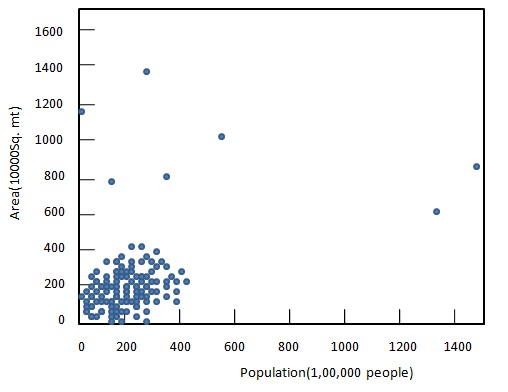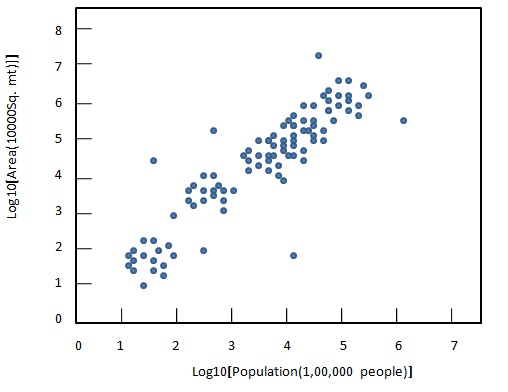# Statistics - Transformations

Data transformation refers to application of a function to each item in a data set. Here $x_i$ is replaced by its transformed value $y_i$ where $y_i = f(x_i)$. Data transformations are carried out generally to make appearance of graphs more interpretable.

There are four major functions used for transformations.

• $log x$ - logarithm transformations. For example sound units are in decibels and is generally represented using log transformations.

• $\frac{1}{x}$ - Reciprocal Transformations. For example Time to complete race/ task is represents using speed. More the speed lesser the time taken.

• $\sqrt{x}$ - Square root Transformations. For example areas of circular ground are compared using their radius.

• ${x^2}$ - Power Transformations. For example to compare negative numbers.

logarithm and Square root Transformations are used in case of positive numbers where as Reciprocal and Power Transformations can be used in case of both negative as well as positive numbers.

Following diagrams illustrates the use of logarithm transformation to compare population graphically.

Before transformationAfter transformation